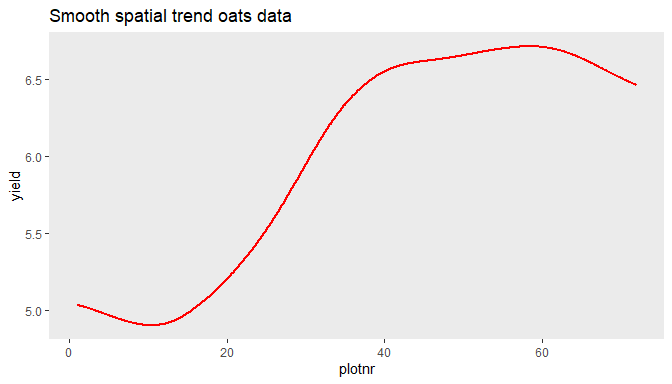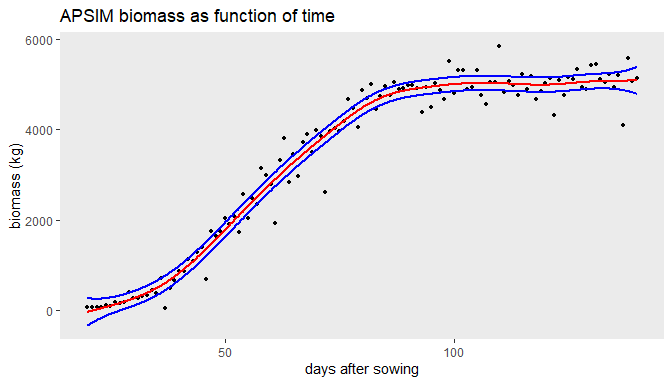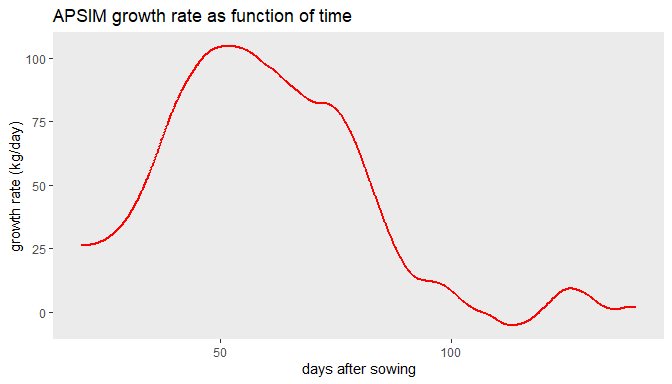# Solving Linear Mixed Models using LMMsolver

#### 2023-11-27

library(LMMsolver)
library(ggplot2)

# The LMMsolver package

The aim of the LMMsolver package is to provide an efficient and flexible system to estimate variance components using restricted maximum likelihood or REML , for models where the mixed model equations are sparse . An example of an application is using splines to model spatial or temporal trends. Another example is mixed model Quantitative Trait Locus (QTL) analysis for multiparental populations, allowing for heterogeneous residual variance and design matrices with Identity-By-Descent (IBD) probabilities .

A Linear Mixed Model (LMM) has the form

$y = X \beta + Z u + e, \quad u \sim N(0,G), \quad e \sim N(0,R)$ where $$y$$ is a vector of observations, $$\beta$$ is a vector with the fixed effects, $$u$$ is a vector with the random effects, and $$e$$ a vector of random residuals. $$X$$ and $$Z$$ are design matrices.

The LMMsolve package can fit models where the matrices $$G^{-1}$$ and $$R^{-1}$$ are a linear combination of precision matrices $$Q_{G,i}$$ and $$Q_{R,i}$$: $G^{-1} = \sum_{i} \psi_i Q_{G,i} \;, \quad R^{-1} = \sum_{i} \phi_i Q_{R,i}$ where the precision parameters $$\psi_i$$ and $$\phi_i$$ are estimated using REML. For most standard mixed models $$1/{\psi_i}$$ are the variance components and $$1/{\phi_i}$$ the residual variances. We use a formulation in terms of precision parameters to allow for non-standard mixed models using tensor product splines introduced in Rodríguez-Álvarez et al. (2015).

If the matrices $$G^{-1}$$ and $$R^{-1}$$ are sparse, the mixed model equations can be solved using efficient sparse matrix algebra implemented in the spam package . To calculate the derivatives of the log-likelihood in an efficient way, the automatic differentiation of the Cholesky matrix was implemented in C++ using the Rcpp package .

# 1 Smooth trend in one dimension.

## 1.1 Oats field trial

As a first example we will use an oats field trial from the agridat package. There were 24 varieties in 3 replicates, each consisting of 6 incomplete blocks of 4 plots. The plots were laid out in a single row.

## Load data.
data(john.alpha, package = "agridat")
#>   plot rep block gen  yield row col
#> 1    1  R1    B1 G11 4.1172   1   1
#> 2    2  R1    B1 G04 4.4461   2   1
#> 3    3  R1    B1 G05 5.8757   3   1
#> 4    4  R1    B1 G22 4.5784   4   1
#> 5    5  R1    B2 G21 4.6540   5   1
#> 6    6  R1    B2 G10 4.1736   6   1

In the following subsections we will use two methods to correct for spatial trend, to show some of the options of the package.

### 1.1.1 Modelling spatial trend using P-splines

In this subsection we will illustrate how the package can be used to fit mixed model P-splines, for details see Boer, Piepho, and Williams (2020).

In the following mixed model we include rep and gen as fixed effect, and we use spl1D to model a one dimensional P-spline with 100 segments, and the default choice of cubical B-splines and second order differences:

## Fit mixed model with fixed and spline part.
obj1 <- LMMsolve(fixed = yield ~ rep + gen,
spline = ~spl1D(x = plot, nseg = 100),
data = john.alpha)

A high number of segments can be used for splines in one dimension, as the corresponding mixed model equations are sparse, and therefore can be solved fast .

round(deviance(obj1), 2)
#>  49.87

We can obtain a table with effective dimensions (see e.g. Rodríguez-Álvarez et al. (2018)) and penalties (the precision parameters $$\psi_i$$ and $$\phi_i$$) using the summary function:

summary(obj1)
#> Table with effective dimensions and penalties:
#>
#>         Term Effective Model Nominal Ratio Penalty
#>  (Intercept)      1.00     1       1  1.00    0.00
#>          rep      2.00     2       2  1.00    0.00
#>          gen     23.00    23      23  1.00    0.00
#>    lin(plot)      1.00     1       1  1.00    0.00
#>      s(plot)      4.18   103      45  0.09 3431.87
#>     residual     40.82    72      45  0.91   13.28
#>
#>  Total Effective Dimension: 72

The effective dimension gives a good balance between model complexity and fit to the data for the random terms in the model. In the table above the first four terms are fixed effects and not penalized, and therefore the effective dimension is equal to the number of parameters in the model. The splF is the fixed part of the spline, the linear trend. The term s(plot) is a random effect, with effective dimension 4.2, indicating that is important to correct for spatial trend.

The estimated genetic effects are given by the coef function:

genEff <- coef(obj1)$gen head(genEff, 4) #> gen_G01 gen_G02 gen_G03 gen_G04 #> 0.0000000 -0.5699756 -1.5231789 -0.4593992 The first genotype (G01) is the reference, as genotypes were modelled as fixed effect in the model. The smooth trend with the standard errors along the field on a dense grid of 1000 points can be obtained as follows: ## Extract smooth trend from mixed model. plotDat1 <- obtainSmoothTrend(obj1, grid = 1000, includeIntercept = TRUE) head(plotDat1) #> plot ypred se #> 1 1.000000 5.036391 0.2462874 #> 2 1.071071 5.035374 0.2448789 #> 3 1.142142 5.034355 0.2434968 #> 4 1.213213 5.033335 0.2421411 #> 5 1.284284 5.032314 0.2408118 #> 6 1.355355 5.031290 0.2395091 The trend can then be plotted. ## Plot smooth trend. ggplot(data = plotDat1, aes(x = plot, y = ypred)) + geom_line(color = "red", linewidth = 1) + labs(title = "Smooth spatial trend oats data", x = "plotnr", y = "yield") + theme(panel.grid = element_blank())### 1.1.2 Modelling spatial trend using random and ginverse arguments. Another way to correct for spatial trend is using the Linear Variance (LV) model, which is closely connected to the P-splines model . First we need to define the precision matrix for the LV model, see Appendix in Boer, Piepho, and Williams (2020) for details: ## Add plot as factor. john.alpha$plotF <- as.factor(john.alpha$plot) ## Define the precision matrix, see eqn (A2) in Boer et al (2020). N <- nrow(john.alpha) cN <- c(1 / sqrt(N - 1), rep(0, N - 2), 1 / sqrt(N - 1)) D <- diff(diag(N), diff = 1) Delta <- 0.5 * crossprod(D) LVinv <- 0.5 * (2 * Delta + cN %*% t(cN)) ## Add LVinv to list, with name corresponding to random term. lGinv <- list(plotF = LVinv) Given the precision matrix for the LV model we can define the model in LMMsolve using the random and ginverse arguments: ## Fit mixed model with first degree B-splines and first order differences. obj2 <- LMMsolve(fixed = yield ~ rep + gen, random = ~plotF, ginverse = lGinv, data = john.alpha) The deviance for the LV-model is 54.49 and the variances summary(obj2, which = "variances") #> Table with variances: #> #> VarComp Variance #> plotF 0.01 #> residual 0.06 as reported in Boer, Piepho, and Williams (2020), Table 1. ## 1.2 Model biomass as function of time. In this section we show an example of mixed model P-splines to fit biomass as function of time. As an example we use wheat data simulated with the crop growth model APSIM. This data set is included in the package. For details on this simulated data see Bustos-Korts et al. (2019). data(APSIMdat) head(APSIMdat) #> env geno das biomass #> 1 Emerald_1993 g001 20 65.57075 #> 2 Emerald_1993 g001 21 60.70499 #> 3 Emerald_1993 g001 22 74.06247 #> 4 Emerald_1993 g001 23 63.73951 #> 5 Emerald_1993 g001 24 101.88005 #> 6 Emerald_1993 g001 25 96.84971 The first column is the environment, Emerald in 1993, the second column the simulated genotype (g001), the third column is days after sowing (das), and the last column is the simulated biomass with medium measurement error. The model can be fitted with obj2 <- LMMsolve(biomass ~ 1, spline = ~spl1D(x = das, nseg = 200), data = APSIMdat) The effective dimensions are: summary(obj2) #> Table with effective dimensions and penalties: #> #> Term Effective Model Nominal Ratio Penalty #> (Intercept) 1.00 1 1 1.00 0.00 #> lin(das) 1.00 1 1 1.00 0.00 #> s(das) 6.56 203 119 0.06 0.01 #> residual 112.44 121 119 0.94 0.00 #> #> Total Effective Dimension: 121 The fitted smooth trend can be obtained as explained before, with standard error bands in blue: plotDat2 <- obtainSmoothTrend(obj2, grid = 1000, includeIntercept = TRUE) ggplot(data = APSIMdat, aes(x = das, y = biomass)) + geom_point(size = 1.2) + geom_line(data = plotDat2, aes(y = ypred), color = "red", linewidth = 1) + geom_line(data = plotDat2, aes(y = ypred-2*se), col='blue', linewidth = 1) + geom_line(data = plotDat2, aes(y = ypred+2*se), col='blue', linewidth = 1) + labs(title = "APSIM biomass as function of time", x = "days after sowing", y = "biomass (kg)") + theme(panel.grid = element_blank())The growth rate (first derivative) as function of time can be obtained using deriv = 1 in function obtainSmoothTrend: plotDatDt <- obtainSmoothTrend(obj2, grid = 1000, deriv = 1) ggplot(data = plotDatDt, aes(x = das, y = ypred)) + geom_line(color = "red", linewidth = 1) + labs(title = "APSIM growth rate as function of time", x = "days after sowing", y = "growth rate (kg/day)") + theme(panel.grid = element_blank())# 3 QTL mapping with IBD probabilities. In QTL-mapping for multiparental populations the Identity-By-Descent (IBD) probabilities are used as genetic predictors in the mixed model . The following simulated example is for illustration. It consists of three parents (A, B, and C), and two crosses AxB, and AxC. AxB is a population of 100 Doubled Haploids (DH), AxC of 80 DHs. The probabilities, pA, pB, and pC, are for a position on the genome close to a simulated QTL. This simulated data is included in the package. ## Load data for multiparental population. data(multipop) head(multipop) #> cross ind pA pB pC pheno #> 1 AxB AxB0001 0.17258816 0.82741184 0 9.890637 #> 2 AxB AxB0002 0.82170793 0.17829207 0 6.546568 #> 3 AxB AxB0003 0.95968439 0.04031561 0 7.899249 #> 4 AxB AxB0004 0.96564081 0.03435919 0 4.462866 #> 5 AxB AxB0005 0.04838734 0.95161266 0 5.207757 #> 6 AxB AxB0006 0.95968439 0.04031561 0 5.265580 The residual (genetic) variances for the two populations can be different. Therefore we need to allow for heterogeneous residual variances, which can be defined by using the residual argument in LMMsolve: ## Fit null model. obj4 <- LMMsolve(fixed = pheno ~ cross, residual = ~cross, data = multipop) dev4 <- deviance(obj4) The QTL-probabilities are defined by the columns pA, pB, pC, and can be included in the random part of the mixed model by using the group argument: ## Fit alternative model - include QTL with probabilities defined in columns 3:5 lGrp <- list(QTL = 3:5) obj5 <- LMMsolve(fixed = pheno ~ cross, group = lGrp, random = ~grp(QTL), residual = ~cross, data = multipop) dev5 <- deviance(obj5) The approximate $$-log10(p)$$ value is given by ## Deviance difference between null and alternative model. dev <- dev4 - dev5 ## Calculate approximate p-value. minlog10p <- -log10(0.5 * pchisq(dev, 1, lower.tail = FALSE)) round(minlog10p, 2) #>  8.76 The estimated QTL effects of the parents A, B, and C are given by: coef(obj5)$QTL
#>     QTL_pA     QTL_pB     QTL_pC
#> -1.2676362  0.6829275  0.5847088